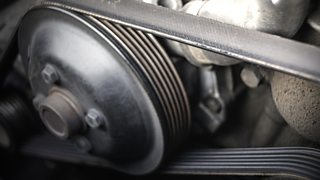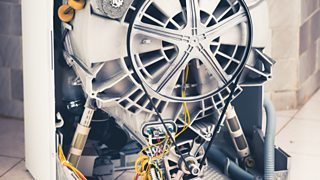# Pulleys and belts

## Pulleys

use , similar to levers, to lift up loads. Pulleys are wheel shaped with a groove that allows a cord to sit inside the groove. They can be used by hand or attached to a motorised to increase the amount of weight that can be lifted.

Pulleys are a simple and manoeuvrable way to move large objects. They are easy to transport to where they are needed and set up, but they do require somewhere stable to hang.

A single pulley changes the direction of force, making pulling down easier than lifting up. Single pulley systems are demonstrated in cranes, lifting a bucket from a well, raising a flag or adjusting window blinds. Even though there is no actual mechanical advantage with one pulley, it is referred to as having a mechanical advantage of one.

A single fixed pulley has a mechanical advantage of one

One pulley doesn’t make a mechanical advantage, as the same amount of force is needed. However, if additional pulleys are added, a mechanical advantage is created. Using two pulleys together means you need half the force to lift. This is called a , and is used to lift large, difficult-shaped objects, such as furniture. Adding more wheels to the block and tackle increases the load it can lift.

A block and tackle
The mechanical advantage is equal to the number of sections of rope pulling up on the object.
A movable pulley

The mechanical advantage of a movable pulley (one where the pulley can move freely along the rope) is two.

### Example

The 10 N load below would still require 10 N of force to lift as the extra pulley is not taking any additional strain in weight - the weight is still taken by only one section of rope.

The 10 N load below would require half of the force to lift. There are two sections of rope taking the strain, so 5 N of force would be needed to lift it. The mechanical advantage would be 2.

Question

The pulley system below features 300 N of load and three pulleys:

What weight is needed to pull the load?

Three sections of rope are taking the strain of the load.

Weight = 300 ÷ 3

= 100 N

## Belts

transfer movement from one rotating pulley to another, each held on a . Shafts and pulley wheels can be made out of any material, whereas pulley belts are generally made from a soft, flexible material such as rubber. Grooves on the pulleys and belts help them to grip and turn.Grooves on a pulley and belt help this grip

Winches, treadmills and washing machines are examples of belt-driven .Washing machine belt drive

Belts can be attached around different-sized pulleys to drive shafts to change speed. As with gears, the bigger the wheel, the slower the speed. The between two pulleys can be calculated.

Velocity ratio = diameter of the driven pulley ÷ diameter of the driver pulley

This can then be used to calculate the output speed.

Output speed = input speed ÷ velocity ratio

### Example

A driven pulley has a diameter of 120 mm and a driver pulley has a diameter of 40 mm.

Velocity ratio = diameter of the driven pulley ÷ diameter of the driver pulley

= 120 ÷ 40 = 3 or 3:1

The smaller driver pulley turns three times to make the driven pulley turn once.

The output speed of the larger driven pulley can then be calculated using the information available - the input speed is 100 revolutions per minute (rpm) and the velocity ratio has been calculated as 3.

Output speed = input speed ÷ velocity ratio

= 100 ÷ 3 = 33 rpm

Question

Calculate the velocity ratio and the output speed of the driven pulley on this lawnmower belt and pulley:

Velocity ratio = diameter of the driven pulley ÷ diameter of the driver pulley

= 15 ÷ 150 = 0.1 or 1:10

The smaller driver pulley turns ten times for every one turn of the driven pulley.

Output speed = input speed ÷ velocity ratio

= 300 ÷ 0.1 = 3,000 rpm

The smaller driven pulley will turn at 3,000 rpm. This is the speed that the lawnmower blade will spin at on the lawnmower, ten times faster than the speed of the motor.

Move on to Test